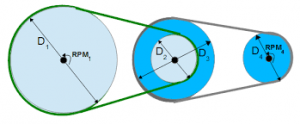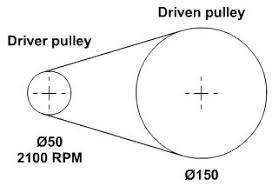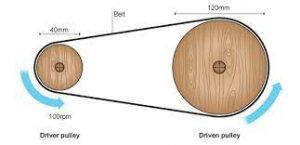## How to Calculate and Solve for r.p.m of Driver Pulley | Pulley Size CalculationsThe image above represents r.p.m of driver pulley.

To compute for r.p.m of driver pulley, three essential parameters are needed and these parameters are Diameter of Driven Pulley (Db), r.p.m. of Driven Pulley (rb) and Diameter of Driver Pulley (Db).

The formula for calculating r.p.m of driver pulley:

ra = (Db x rb/Da

Where:

ra = r.p.m. of Driver Pulley
Db = Diameter of Driven Pulley
rb = r.p.m. of Driven Pulley
Da = Diameter of Driver Pulley

Let’s solve an example;
Find the r.p.m of driver pulley when the diameter of driven pulley is 21, the r.p.m of driven pulley is 18 and the diameter of driver pulley is 14.

This implies that;

Db = Diameter of Driven Pulley = 21
rb = r.p.m. of Driven Pulley = 18
Da = Diameter of Driver Pulley = 14

ra = (Db x rb/Da
ra = (21 x 18)/14
ra = 378/14
ra = 27

Therefore, the r.p.m of driver pulley is 27 Hz.

## How to Calculate and Solve for r.p.m of Driven Pulley | Pulley Size CalculationsThe image above represents r.p.m of driven pulley.

To compute for r.p.m of driven pulley, three essential parameters are needed and these parameters are Diameter of Driven Pulley (Db), r.p.m. of Driver Pulley (ra) and Diameter of Driver Pulley (Db).

The formula for calculating r.p.m of driven pulley:

rb = (Da x ra/Db

Where:

rb = r.p.m. of Driven Pulley
Da = Diameter of Driver Pulley
ra = r.p.m. of Driver Pulley
Db = Diameter of Driven Pulley

Let’s solve an example;
Given that the diameter of driver pulley is 10, the r.p.m of driver pulley is 4 and the diameter of driven pulley is 6. Find the r.p.m of driven pulley?

This implies that;

Da = Diameter of Driver Pulley = 10
ra = r.p.m. of Driver Pulley = 4
Db = Diameter of Driven Pulley = 6

rb = (Da x ra/Db
rb = (10 x 4)/6
rb = 40/6
rb = 6.67

Therefore, the r.p.m of driven pulley is 6.67 Hz.

## How to Calculate and Solve for Diameter of Driver Pulley | Pulley Size CalculationsThe image above represents diameter of driver pulley.

To compute for diameter of driver pulley, three essential parameters are needed and these parameters are Diameter of Driven Pulley (Db), r.p.m. of Driver Pulley (ra) and r.p.m. of Driven Pulley (rb).

The formula for calculating diameter of driver pulley:

Da = (Db x rb/ra

Where:

Da = Diameter of Driver Pulley
Db = Diameter of Driven Pulley
rb = r.p.m. of Driven Pulley
ra = r.p.m. of Driver Pulley

Let’s solve an example;
Find the diameter of driver pulley when the diameter of driven pulley is 12, the r.p.m of driven pulley is 24 and the r.p.m of driver pulley is 10.

This implies that;

Db = Diameter of Driven Pulley = 12
rb = r.p.m. of Driven Pulley = 24
ra = r.p.m. of Driver Pulley = 10

Da = (Db x rb/ra
Da = (12 x 24)/10
Da = 288/10
Da = 28.8

Therefore, the diameter of driver pulley is 28.8 m.